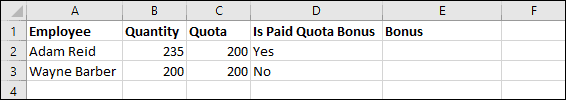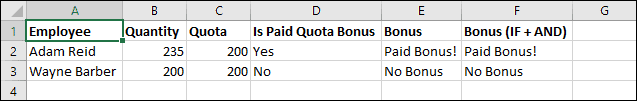# Excel IF formulas with multiple arguments?

## What is the IF function?

The IF function is an Excel function that is used to test for a logical condition and return different values depending upon it.

For example you could test if cell A1 contains the number 1 and return “Yes” if it does using this formula:

=IF(A1=1,”Yes,”No”)

If you’re unfamiliar with Excel formulas and functions you could greatly benefit from our free Basic Skills E-book.
Advanced functions such as IF are covered in depth in our Expert Skills Books and E-books.

## The problem with nested IF functions

Sometimes you need to test for more than one condition at the same time. Consider this example:In this example you want to use an IF function to determine whether employees are paid a bonus or not. Only employees who have exceeded their quota and are paid a quota bonus should receive a bonus.

You could solve this problem using ‘nested’ IF functions. Nesting means placing one function inside another function. The formula would look like this:

=IF(B2>C2,IF(D2=”Yes”,”Paid Bonus!”,”No Bonus”),”No Bonus”)

If this formula doesn’t already look complicated to you, imagine how it would look with 3 nested IF functions or even more!

Nesting functions isn’t necessarily incorrect, but it tends to make formulas harder to understand and more prone to error.

## Simplifying the formula with the AND function

Excel’s AND function allows you to test for multiple conditions within a single function. Here’s how the formula looks using the AND function instead of two nested IF functions.

=IF(AND(B2>C2,D2=”Yes”),”Paid Bonus!”,”No Bonus”)

This has greatly simplified the formula, and is the correct approach if you need to return a single result in response to multiple conditions. Excel also contains an OR function that returns a result if any one of the conditions within it are true.

AND and OR are explained in much greater depth in our Expert Skills Books and E-books.

## Sample workbook